### Home > CCAA > Chapter 9 Unit 10 > Lesson CC3: 9.2.3 > Problem9-91

9-91.

Copy and complete each of the Diamond Problems below. The pattern used in the Diamond Problems is shown at right.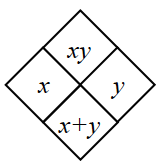1.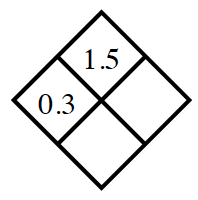What is $1.5$ divided by $0.3$?

1.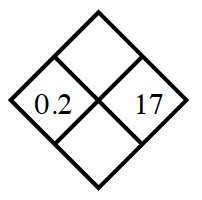Add $x$ and $y$ to fill the lower box.
Multiply them to fill the upper box.

1.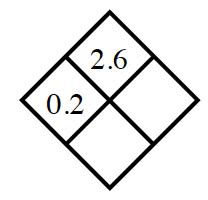What times $0.2$ is equal to $2.6$?

$y = 13$
Can you find the sum of $0.2$ and $13$?

1.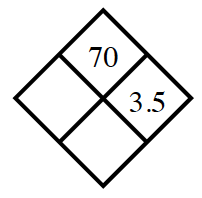This problem is very similar to part (c).